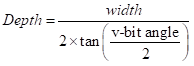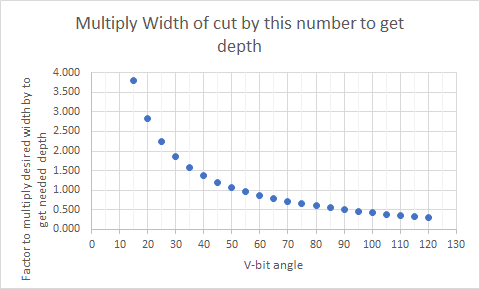# V bit depth of cut

I am looking for the math formula that will tell me what the depth of cut will be between two vectors using any given V bit. I have seen a YouTube video on this, but it was too far over my head to understand. I guess what I need is the formula and a corresponding example.

Thanks in advance for any help.

It’s geometry/trigonometry — Pythagoras’ Theorem.

Alternately, just draw the tool in profile and a rectangle of the desired width/thickness.

Will: I know that A squared + B squared = C squared. However, with the problem, as I understand it, I only know B. Sides A and C remain unknown. So, I only know side, B and its opposing angle (1/2 of the V bit in use). There is some calculus in there that can figure that out, but I don’t have that. Bottom line, I’m still lost.

If I understand what you are asking for is: For a feature of a particular width what depth do I need to use for a particular angle V-bit? If that is correct the formula you want should beBut since this should give a constant ratio between depth and width for each particular angle v-bit here is the calculation:

V bit angle Multiply Width of cut by this number to get depth
5 11.452
10 5.715
15 3.798
20 2.836
25 2.255
30 1.866
35 1.586
40 1.374
45 1.207
50 1.072
55 0.960
60 0.866
65 0.785
70 0.714
75 0.652
80 0.596
85 0.546
90 0.500
95 0.458
100 0.420
105 0.384
110 0.350
115 0.319
120 0.289John

5 Likes

Specifically, you know two values:

• diameter to which you wish to cut
• V angle

I always look this sort of thing up at:

https://www.mathportal.org/calculators/plane-geometry-calculators/right-triangle-calculator.php

so assume the V endmill is on its side, and half its angle equals angle alpha and the dimension a == half the width which we wish to cut to, so we are trying to determine length b — given for an example:

• 60 degree V endmill
• cutting to a width of 0.75"

We need to enter the values:

• alpha == 30 degrees
• a == 0.375"

and

b == 3/8" / tan(30 degrees)

tan of 30 degrees == 0.577350269

so we EDIT: correction divide (not multiply):

3/8" / 0.577350269

to get:

0.649519053

for the depth which we need to cut to.

For an example of drawing things up in profile and just setting the depth thus see

2 Likes

I think you want to divide here.

Width of cut / 2 / tan(half angle of bit)

Here’s a post I wrote explaining the math, but looking for the width. We just change the equation.

3 Likes

John: That’s not quite what I asked . . . I think. Maybe an example would be better. So, if I have two vectors that are .05 inches apart, and I have a 90 degree V bit, what is the dept to which the V bit will carve.

I’m very certain those replies are what you’re looking for. Using Stankus table (I’m saving that) I’m able to confirm Wills example. they both give the same values. 0.05" gap is not a lot and a 90deg vbit will not cut very deep between them. What is the end result you’re looking for? because with those conditions you’ll have a very shallow 0.025" deep carve.

1 Like

(A quick edit since I was off by a factor of two)
So are your running along each vector with the v-bit and wanting to know how high the ridge is between them? Two parallel v-bit paths with make a W shape with the middle of the W’s height dependent on the spacing (this height can be calculated from the bottom of the W easiest).
It would use the calculations I provided. Take the spacing between the two vectors.and multiply by the factor in my previous post. That should give you the height of the ridge relative to the BOTTOM of the v-bit groove. For your example of 0.05 inch spacing and a 90 deg v-bit. The factor above for a 90 deg v-bit is 0.500, so the height of the ridge between the v-bit valleys is 0.05” X0.500 which should be 0.025”

General form (contingent of cut being deep enough for the tool paths to intersect)
Height of ridge=(spacing) X (V-bit factor)

If that isn’t what you are asking, a picture might help.

John

Will: That’s what I was looking for. Thanks.

John: My apologies. You were right and I was wrong. The value your table supplies is the same results that Will’s web page shows. I do have a followup. I’m not the greatest math guy and I tried to write a formula in Excel using what you supplied. The formula looks like: =B6/2*TAN((B7/2)) where B6 is width or distance between two vectors, and B7 is the angle of the V bit. When I input .05 in B6 (width between two vectors) and 90 for the value in B7, the angle of the V bit, the result I get is .040494, but according the web page and your calculations it should be .05. There must be an error in my formula and am wondering if you can spot it?

Thanks

(edit cause there is one more issue here)
Just an order of operations issue.
Try =b6/(2*tan(b7/2))

The excel trig functions use radians for the angle, so if using degrees the formula would be
=b6/(2tan(b7pi()/360))

My initial answer did not do the conversion from degrees to radians

John

2 Likes

Or…
=B6/2/TAN((B7/2))

This one actually works…=(B6/2)*TAN(RADIANS(B7/2))

2 Likes

And THIS one works correctly:2 Likes

weird thing when I edited my prior post to correct the radians the multply sign disappeared in the corrected formula (but not the original version in radians)

1 Like

Right you are! (Teamwork)

1 Like

Thank you for the help with the formula. I’m a happy camper now. Blessings to all.

This topic was automatically closed 30 days after the last reply. New replies are no longer allowed.